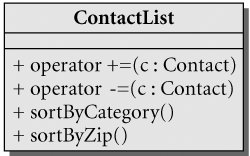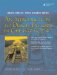# Generics, Algorithms, and Operators

Overloading operator symbols makes it possible to define a common interface for our classes that is consistent with that of the basic types. Many generic algorithms take advantage of this by using the common operators to perform basic functions such as comparison.

The qSort() function is a generic algorithm that is implemented using the heap sort algorithm. In Example 10.7, we show how it can be used on two similar but different containers.

qSort() can be applied to any Qt container of objects that have publicly defined functions operator<() and operator==(). Containers of built-in numeric types can also be sorted with this function.

Example 10.7. src/collections/sortlist/sortlist4.cpp

 ```#include #include #include // for qSort() #include // for cin and cout using namespace qstd; class CaseIgnoreString : public QString { public: CaseIgnoreString(const QString& other = QString()) : QString(other) {} bool operator<(const QString & other) const { return toLower() < other.toLower(); } bool operator==(const QString& other) const { return toLower() == other.toLower(); } }; int main() { CaseIgnoreString s1("Apple"), s2("bear"), s3 ("CaT"), s4("dog"), s5 ("Dog"); ASSERT_TRUE(s4 == s5); ASSERT_TRUE(s2 < s3); ASSERT_TRUE(s3 < s4); QList namelist; namelist << s5 << s1 << s3 << s4 << s2; <-- 1 qSort(namelist.begin(), namelist.end()); int i=0; foreach (QString stritr, namelist) { cout << QString("namelist[%1] = %2") .arg(i++).arg(stritr) << endl; } QStringList strlist; strlist << s5 << s1 << s3 << s4 << s2; <-- 2 qSort(strlist.begin(), strlist.end()); cout << "StringList sorted: " + strlist.join(", ") << endl; return 0; } ```   (1)Insert all items in an order that is definitely not sorted. (2)The value collection holds QString, but we are adding CaseIgnoreString. A conversion is required.

operator<<(), which is the left shift operator from C, has been overloaded in the QList class to append items to the list.

Example 10.8 shows the output of this program.

Example 10.8. src/collections/sortlist/sortlist-output.txt

 ```namelist = Apple namelist = bear namelist = CaT namelist = dog namelist = Dog StringList sorted: Apple, CaT, Dog, bear, dog ```

Notice that the sorting order is case sensitive when we add CaseIgnoreString objects to a QStringList. This is because a CaseIgnoreString must be converted into a QString as it is added to strlist. Therefore, when strlist's elements are compared, they are compared as QStrings.

Exercises: Generics, Algorithms, and Operators

 1 A QStringList is a value container of objects that have lazy copy-on-write. In a way, it is like a pointer-collection, but smarter. In Example 10.7, a CaseIgnoreString was added to a QStringList, which required a conversion. Does this require a copy of the actual string data? Why or why not? 2 Add some more functions to ContactList:Operators += and -= should add() and remove() respectively. Write some client code that tests these functions.

### Serializer PatternAn Introduction to Design Patterns in C++ with Qt 4
ISBN: 0131879057
EAN: 2147483647
Year: 2004
Pages: 268